Quantitative MCQ - 16

# Quantitative MCQ - 16

Test Description

## 30 Questions MCQ Test Quantitative Aptitude for Competitive Examinations | Quantitative MCQ - 16

Quantitative MCQ - 16 for Quant 2022 is part of Quantitative Aptitude for Competitive Examinations preparation. The Quantitative MCQ - 16 questions and answers have been prepared according to the Quant exam syllabus.The Quantitative MCQ - 16 MCQs are made for Quant 2022 Exam. Find important definitions, questions, notes, meanings, examples, exercises, MCQs and online tests for Quantitative MCQ - 16 below.
Solutions of Quantitative MCQ - 16 questions in English are available as part of our Quantitative Aptitude for Competitive Examinations for Quant & Quantitative MCQ - 16 solutions in Hindi for Quantitative Aptitude for Competitive Examinations course. Download more important topics, notes, lectures and mock test series for Quant Exam by signing up for free. Attempt Quantitative MCQ - 16 | 30 questions in 30 minutes | Mock test for Quant preparation | Free important questions MCQ to study Quantitative Aptitude for Competitive Examinations for Quant Exam | Download free PDF with solutions
 1 Crore+ students have signed up on EduRev. Have you?
Quantitative MCQ - 16 - Question 1

### Direction (Q1-5): Study the following line graph carefully to answer the questions based on it. The graph shows the amount lent by Mohit and Arunoday to six persons.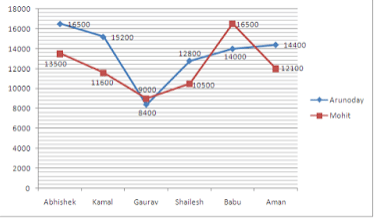What is the difference between the average amount lent out by Arunoday and Mohit?

Detailed Solution for Quantitative MCQ - 16 - Question 1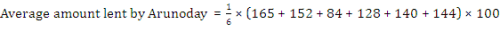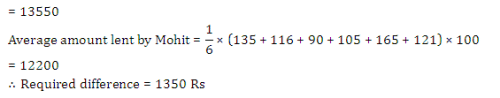Quantitative MCQ - 16 - Question 2

### Direction (Q1-5): Study the following line graph carefully to answer the questions based on it. The graph shows the amount lent by Mohit and Arunoday to six persons.What is the interest earned by Arunday from Abishek and Shailesh if he lent them money at 10% and 11% p.a. respectively, interest being compound annually for 2 years?

Detailed Solution for Quantitative MCQ - 16 - Question 2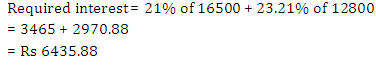Quantitative MCQ - 16 - Question 3

### Direction (Q1-5): Study the following line graph carefully to answer the questions based on it. The graph shows the amount lent by Mohit and Arunoday to six persons.Babu returned Rs 16633.4 to Arunday after 2 years. Find the rate pcpa at which he borrowed the amount at compound interest compounded annually?

Detailed Solution for Quantitative MCQ - 16 - Question 3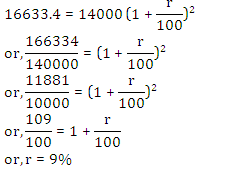Quantitative MCQ - 16 - Question 4

Direction (Q1-5): Study the following line graph carefully to answer the questions based on it.

The graph shows the amount lent by Mohit and Arunoday to six persons.If Saket borrowed same amount as Babu from Arunday at same rate of interest for 3 years at simple interest while Babu borrowed at compound interest compounded annually at same rate and time period If the difference between their interest is Rs 992.25 then find the rate pcpa.

Detailed Solution for Quantitative MCQ - 16 - Question 4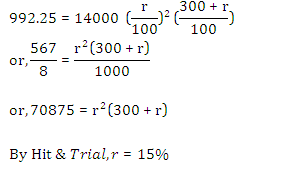Quantitative MCQ - 16 - Question 5

Direction (Q1-5): Study the following line graph carefully to answer the questions based on it.

The graph shows the amount lent by Mohit and Arunoday to six persons.Find the amount earned by Mohit by lending money to given persons at 10% pa in 1 year?

Detailed Solution for Quantitative MCQ - 16 - Question 5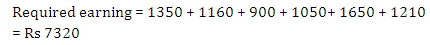Quantitative MCQ - 16 - Question 6

The compound interest on a certain sum for 2 years is Rs. 756 and Simple interest is Rs. 720. If the sum is invested such that the SI is Rs. 900 and the number of years is equal to the rate percent per annum, find the rate percent?

Detailed Solution for Quantitative MCQ - 16 - Question 6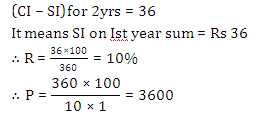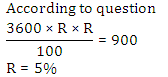Quantitative MCQ - 16 - Question 7

Arun and Gaurav have to clear their respective loans by paying 3 equal annual instalments of Rs. 30,000 each. Arun pays @ 10% per annum of simple interest while Gaurav pays 10% per annum compound interest. What is the difference in their loan amounts?

Detailed Solution for Quantitative MCQ - 16 - Question 7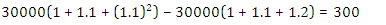Quantitative MCQ - 16 - Question 8

Anjali invested an amount of Rs. 5800 for 2 years. At what rate of Compound Intrest will she get an amount of Rs. 594.50 at the end of two years?

Detailed Solution for Quantitative MCQ - 16 - Question 8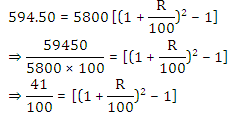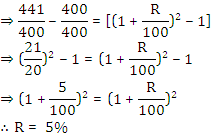Quantitative MCQ - 16 - Question 9

A man borrows Rs. 3000 at the rate of 10%  compounded annually. At the end of each year, he pays back Rs. 1000. How much amount should he pay at the end of the 3rd year to clear all his dues?

Detailed Solution for Quantitative MCQ - 16 - Question 9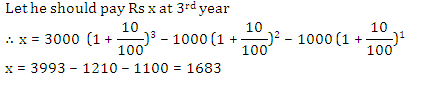Quantitative MCQ - 16 - Question 10

Anjali took a loan of Rs. 20,000 to purchase a TV set from LG. She promised to make the payment after three years. The company charges CI @ 10% per annum for the same. But, suddenly the company announces the rate of interest as 15% per annum for the last one year of the loan period. What extra amount she has to pay due to the announcement of the new rate of interest?

Detailed Solution for Quantitative MCQ - 16 - Question 10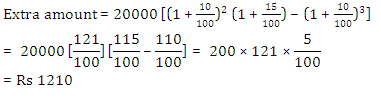Quantitative MCQ - 16 - Question 11

A sum of money was invested for 14  years in scheme A which offers simple intrest @8% per annum.  The amount received from Scheme A after 14 years was then invested for two years in Scheme B which offers CI @ 10% per annum. If the intrest received from Scheme B was Rs. 6678, then what was the sum invested in Scheme A?

Detailed Solution for Quantitative MCQ - 16 - Question 11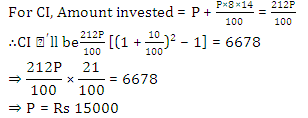Quantitative MCQ - 16 - Question 12

Equal sums of money are deposited in two different banks by ABC  Enterprises, one at compound interest,  compounded annually and the other at simple interest, both at 5% per annum. If after two years, the difference in the amounts comes to Rs. 200, what are the amounts deposited with each bank?

Detailed Solution for Quantitative MCQ - 16 - Question 12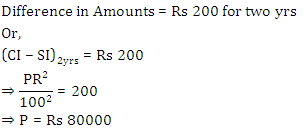Quantitative MCQ - 16 - Question 13

The ratio of CI and SI for two years is 3 : 2 while sum invested is Rs. 5000. What will be the difference between the CI and SI earned after three years?

Detailed Solution for Quantitative MCQ - 16 - Question 13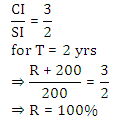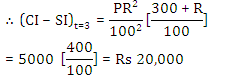Quantitative MCQ - 16 - Question 14

P and Q invest some amount under SI and CI respectively but for the same period at 6% per annum. Each gets a total amount of Rs. 65,000 at the end of 6 years. Which of the following is definitely true?(i) Q’s initial principal is less than that of P(ii) Q’s initial principal is equal to that of P(iii) P’s percentage earning is less than that of Q

Detailed Solution for Quantitative MCQ - 16 - Question 14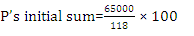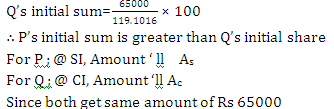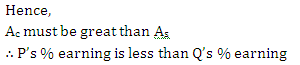Quantitative MCQ - 16 - Question 15

A  depositsRs. 20,000 in a private company at the rate of 16% compounded annually whereas B deposits an equal Sum in PNB at the rate of 15% compounded half-yearly. If both deposit their money for 3/2 years only, then the intrest A will earn is how much percent more or less than the intrest earn by B?

Detailed Solution for Quantitative MCQ - 16 - Question 15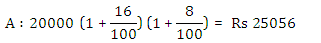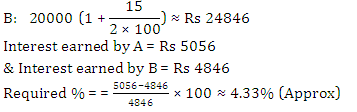Quantitative MCQ - 16 - Question 16

What amount of compound interest can be obtained on an amount of Rs 8840 at the rate of 5% per annum at the end of 3 yr?

Detailed Solution for Quantitative MCQ - 16 - Question 16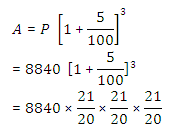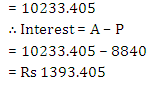Quantitative MCQ - 16 - Question 17

A tree’s height increases annually by 1/8th of its height. By how much will it’s height increase after 2.5 years if it stands today at 10 m height?

Detailed Solution for Quantitative MCQ - 16 - Question 17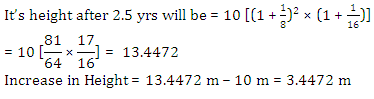Quantitative MCQ - 16 - Question 18

CI on a certain sum for 2 years is Rs. 40.80 and SI  is Rs. 40 for same year. What will be the rate of interest?

Detailed Solution for Quantitative MCQ - 16 - Question 18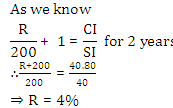Quantitative MCQ - 16 - Question 19

A sum of Rs. 18750 is left by will by a father to be divided between two sons, 12 and 14 years of age, so that when they attain maturity at 18,  the amount(P + I)  received by each at 5% simple interest will be the same. Find the sum allotted at present to the younger son.

Detailed Solution for Quantitative MCQ - 16 - Question 19

Let younger son get x at present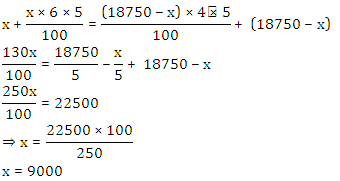Quantitative MCQ - 16 - Question 20

Siddhartha has deposited a certain amount in the bank to earn compound interest at 10% per annum. The difference between the interest of the third year and second year on the amount is Rs. 1,10. What amount has Siddhartha deposited?

Detailed Solution for Quantitative MCQ - 16 - Question 20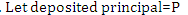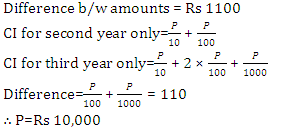Quantitative MCQ - 16 - Question 21

Directions (21-25): Study the following graph carefully to answer these questions.
The line graph shows the population of 2 states over a period of 6 years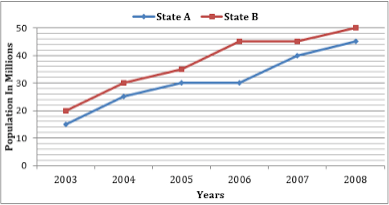For state B the percentage rise in population from the previous year was the highest in which of the following years?

Detailed Solution for Quantitative MCQ - 16 - Question 21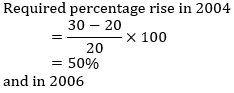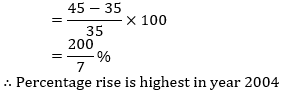Quantitative MCQ - 16 - Question 22

Directions (21-25): Study the following graph carefully to answer these questions.
The line graph shows the population of 2 states over a period of 6 yearsWhat was the average population of state B (in million) for all the years together?

Detailed Solution for Quantitative MCQ - 16 - Question 22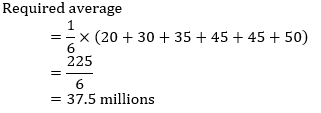Quantitative MCQ - 16 - Question 23

Directions (21-25): Study the following graph carefully to answer these questions.
The line graph shows the population of 2 states over a period of 6 yearsWhat is the percentage rise in population of state A in 2007 from the previous year?

Detailed Solution for Quantitative MCQ - 16 - Question 23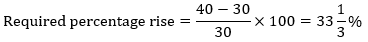Quantitative MCQ - 16 - Question 24

Directions (21-25): Study the following graph carefully to answer these questions.
The line graph shows the population of 2 states over a period of 6 yearsWhat is the ratio between the total populations of states A and B respectively for all the years together?

Detailed Solution for Quantitative MCQ - 16 - Question 24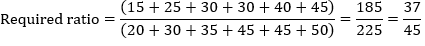Quantitative MCQ - 16 - Question 25

Population of state A in 2005 is what per cent of its total population for all the years together?

Detailed Solution for Quantitative MCQ - 16 - Question 25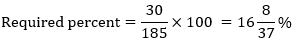Quantitative MCQ - 16 - Question 26

Directions (26-30): what should come in place of the question mark (?) in the following number series?

2, 26, 286, ?, 18018, 90090, 270270

Detailed Solution for Quantitative MCQ - 16 - Question 26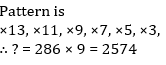Quantitative MCQ - 16 - Question 27

Directions (26-30): what should come in place of the question mark (?) in the following number series?

358, 356, 352, 344, 328, 296, ?

Detailed Solution for Quantitative MCQ - 16 - Question 27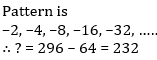Quantitative MCQ - 16 - Question 28

Directions (26-30): what should come in place of the question mark (?) in the following number series?

8, ?, 30, 105, 472.5, 2598.75, 16891.875

Detailed Solution for Quantitative MCQ - 16 - Question 28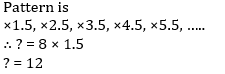Quantitative MCQ - 16 - Question 29

Directions (26-30): what should come in place of the question mark (?) in the following number series?

3, 4, ?, 21, 85, 110, 326

Detailed Solution for Quantitative MCQ - 16 - Question 29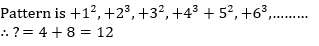Quantitative MCQ - 16 - Question 30

Directions (26-30): what should come in place of the question mark (?) in the following number series?

50000, 10000, 2500, 500, 125, ?, 6.25

Detailed Solution for Quantitative MCQ - 16 - Question 30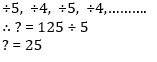## Quantitative Aptitude for Competitive Examinations

33 videos|34 docs|140 tests
 Use Code STAYHOME200 and get INR 200 additional OFF Use Coupon Code
Information about Quantitative MCQ - 16 Page
In this test you can find the Exam questions for Quantitative MCQ - 16 solved & explained in the simplest way possible. Besides giving Questions and answers for Quantitative MCQ - 16, EduRev gives you an ample number of Online tests for practice

## Quantitative Aptitude for Competitive Examinations

33 videos|34 docs|140 tests